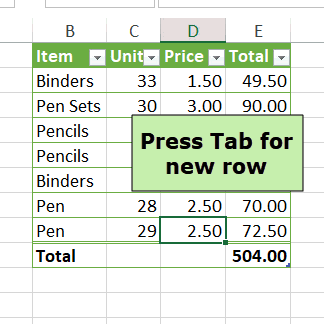## Add Data to Excel Table with Total Row

With a formatted Excel table, you can turn the Total Row on or off easily, and it shows at the bottom of the table. Someone asked me how to add data to Excel Table with Total Row showing – they were hiding the totals every time they wanted to add data. You don’t need to do that!

### Excel Table Total Row

After you create a formatted Excel table, it’s easy to turn the Total Row on or off.

• Select a cell in the Excel table
• On the Excel Ribbon, under Table Tools, click the Design tab
• In the Table Style Options group, add or remove the checkmark for Total Row### Total Row Calculations

When you first add the Total Row, Excel automatically puts a Subtotal formula into the last column of the total row, to show a sum or count of the items in that column.

• If the entries are all numbers, the first argument is 109 – SUM.
• If there are non-numeric entries, the first argument is 103 – COUNTA### Totals for Filtered Data

Excel uses the SUBTOTAL function in the total row, because that function only calculates the total for items that are visible after applying a filter.

You can replace the automatic formula in the Total Row, if you prefer, and you can add Total formulas in other columns.

For example, add an AGGREGATE formula in the Total row — it has more functions and options than SUBTOTAL.### Totals at Bottom or Top?

When you have a list or table on a worksheet, do you like to show the totals above that, or at the bottom?

In my Excel files, the Total row is usually hidden in the formatted Excel Tables. If  totals need to be shown,  I put formulas  above the table, or I build a pivot table on a different sheet, and show the totals there.

### Add Data to Excel Table

With the built-in Total Row for Excel tables, there isn’t an option to show the totals at the top. It’s the bottom, or nothing.

But, if you like to show the Total row, you don’t need to hide that row, when it’s time to add data at the bottom of the table.

Use one of the following methods to add data to Excel Table with Total Row showing. With both methods, the Total row moves down automatically.

To add a single row of new data:

• Select the last cell in the last row of data
• Press the Tab key, to start a new row

This animated screen shot show the Tab method.### Add Multiple Rows of New Data

To paste new data at the end of the table

• Copy the data that you want to add (Ctrl+C)
• Select the first cell in the Title row, even if there’s text or a formula there
• Then, press Enter, or Ctrl+V, to paste the copied data

This animated screen shot show the Copy and Paste method.### More Excel Table Tips

See more Excel Table tips on my Contextures site. There is also a sample workbook to download, so you can test these Table techniques.

And for all you ever wanted to know about Excel Tables, see this book:

Excel Tables: A Complete Guide, by Zack Barresse and Kevin Jones.___________________________

### Add Data to Excel Table with Total Row___________________________